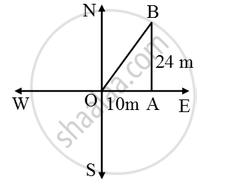# A man goes 10 m due east and then 24 m due north. Find the distance from the starting point - Mathematics

Sum

A man goes 10 m due east and then 24 m due north. Find the distance from the starting point

#### Solution

Let the initial position of the man be O and his final position be B. Since the man goes 10 m due east and then 24 m due north.

Therefore, ∆AOB is a right triangle right-angled at A such that OA = 10 m and AB = 24 m.By Phythagoras theorem, we have

OB^2 = OA^2 + AB^2

⇒ OB^2 = 10^2 + 24^2 = 100 + 576 = 676

⇒ OB = \sqrt { 676 } = 26 m

Hence, the man is at a distance of 26 m from the starting point

Concept: Right-angled Triangles and Pythagoras Property
Is there an error in this question or solution?